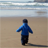# QlikView App Dev

Discussion Board for collaboration related to QlikView App Development.

cancel
Showing results for
Did you mean:MVP

## RE:what wrong in my expression?

hi all,

Am facing the issue in expression can any one correct me?  in pivot table

i dnt hve master calender....

my script lik dis:

PRJ_NUMBER,

PERIOD_NAME,

BALANCE_ACTUAL,

BALANCE_BUDGET,

PERIOD_YEAR,

Year(PERIOD_YEAR) as CYear,

Month(PERIOD_YEAR) as CMonth,

Date(PERIOD_YEAR) as CDate,

PERIOD_NUM,

PRJ_BU

FROM [lib://Management/MANAGEMENT_PACK.QVD]

(qvd);

current month-  Sum({\$<Month = {\$(=Max(Month))},Year = ,Quarter = ,Month = >} BALANCE_ACTUAL)

6months[til jun'15]  --  Sum({<Month = {"<=\$(=max(Month, 6))"},Year = {\$(=(max(Year)-1))}  >}BALANCE_ACTUAL)

Ytd[til dec'15-  Sum({<Year=, Month=, Quarter=, Week=, DateField=, DateNum={">=\$(=Num(YearStart(Max(DateNum))))<=\$(=Max(DateNum))"}>} BALANCE_ACTUAL)

where am geting wrng values so kindly  help me out where am getting problem

thanks

suresh

1 Solution

Accepted SolutionsMaster

hi SURESH,

For Variance % you can take like

(Sum(Curr Actual)-sum(Curr Budget)/(Sum(Curr Actual)+sum(Curr Budget)))*100

Let me know if not

41 RepliesMVP

on a high level , double quotes are missing in the first expression

current month-  Sum({\$<Month = {"(=Max(Month))"},Year = ,Quarter = ,Month = >} BALANCE_ACTUAL)MVP
Author

thanks avinash.

but if do that also doesnt chnge in values? shwng same values

thanks

SureshMVP

can you please share you app and the count your looking for ? because it would be hard to identify the error without seeing the fileChampion

Try this:

=Sum({<Month = {'\$(=Max(Month))'},Year = ,Quarter = ,Month = >} BALANCE_ACTUAL)

Make sure Month is interger as Max(Month) returns integerPartner

Hi

Like this ?

current month-  Sum({\$<DateField = {\$(=addmonths(Max(Month)))},Year = ,Quarter = ,Month = >} BALANCE_ACTUAL)MVP
Author

no luck... nagarjuna, & balraj

giving same valuesChampion

Did you check whether you Month Field is having String or Numeric...

Becoz max(Month) would return an interger..

If you Month Field is having values like Jan, Feb, Mar etc where max is Mar.

Now, IF I would do ,max(Month), I wud get 3 which is not matching with Mar..

Did you get??MVP
Author

i hve like  string only..

like jan,feb...Champion

Then It would not work.

Create a numeric field say, Period_Number at script like in Calender table:

CalenderYear*12+month(CalenderMonth) as Period_Number

and then try..with this new field, it wud surely work